# Precalculus : Graph Logarithms

## Example Questions

### Example Question #1 : Graph Logarithms

What is the domain of the function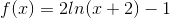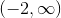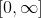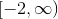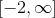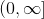Explanation:

The function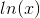is undefined unless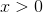. Thus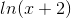is undefined unless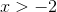because the function has been shifted left.

### Example Question #1 : Graph Logarithms

What is the range of the function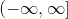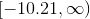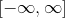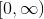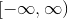Explanation:

To find the range of this particular function we need to first identify the domain. Since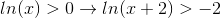we know that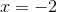is a bound on our function.

From here we want to find the function value asapproaches.

To find this approximate value we will plug in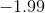into our original function.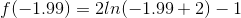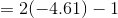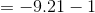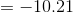This is our lowest value we will obtain. As we plug in large values we get large function values.

Therefore our range is:### Example Question #1 : Graph Logarithms

Which of the following logarithmic functions match the provided diagram?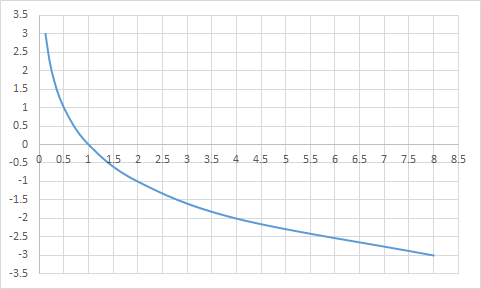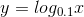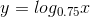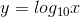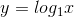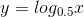Explanation:

Looking at the diagram, we can see that when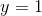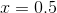. Sincerepresents the exponent andrepresents the product, and any base with an exponent of 1 equals the base, we can determine the base to be 0.5.

### Example Question #101 : Exponential And Logarithmic Functions

Which of the following diagrams represents the graph of the following logarithmic function?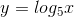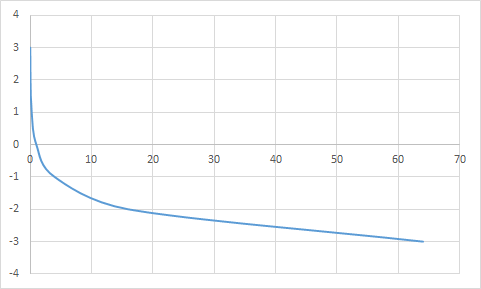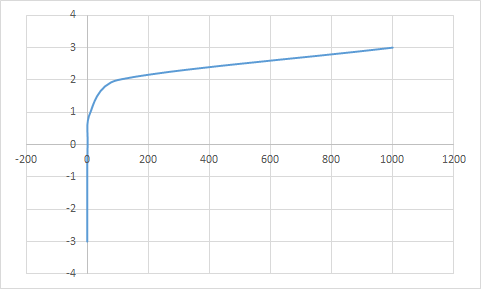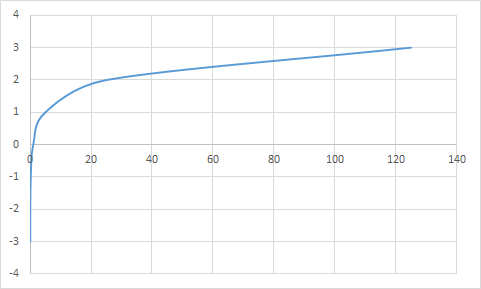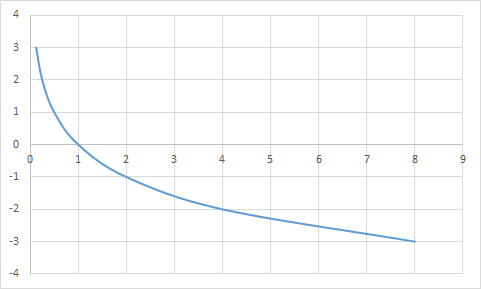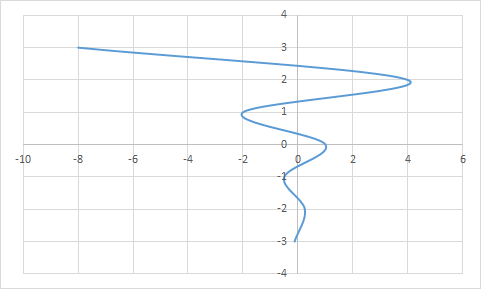Explanation:

For,is the exponent of base 5 andis the product. Therefore, when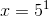and when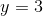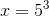. As a result, the correct graph will havevalues of 5 and 125 atand, respectively.

### Example Question #2 : Graph Logarithms

Which of the following diagrams matches the given logarithmic function: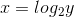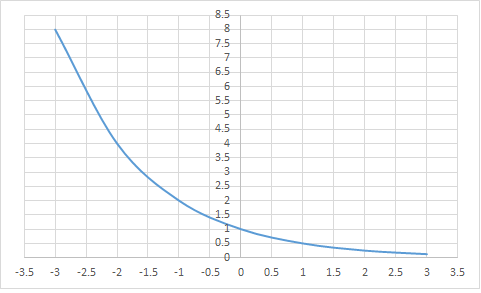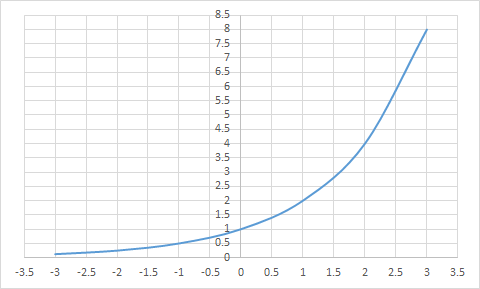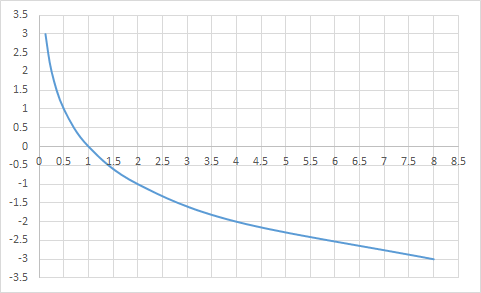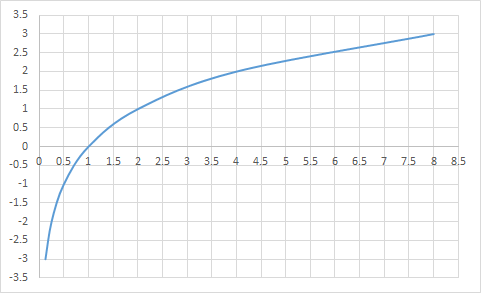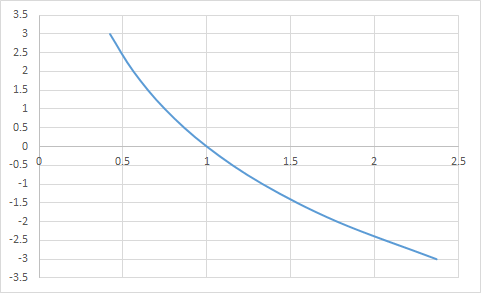Explanation:

For this function,represents the exponent and y represents the product of the base 2 and its exponent. On the diagram, it is clear that as thevalue increases, thevalue increases exponentially and at,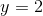. Those two characteristics of the graph indicate that x is the exponent value and the base is equal to 2.

### Example Question #102 : Exponential And Logarithmic Functions

Which of the following logarithmic functions match the given diagram?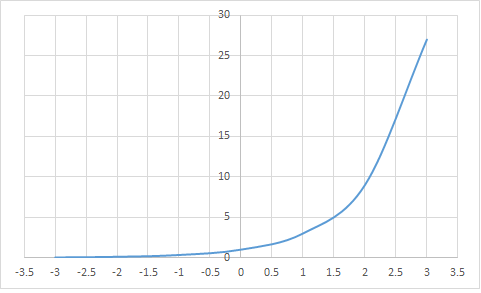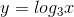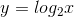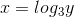Explanation:

Looking at the graph, the y-value diminishes exponentially asdecreases and increases rapidly as the x-value increases, which indicates thatis the exponent value for the equation.

Also,whenand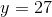when, which can be expressed as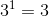and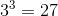, respectively.

This indicates that the diagram is consistent with the function.

### All Precalculus Resources